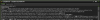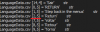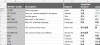# Get_char_at() parser not finding special characters despite being UTF-8

T

#### Teknopants

##### Guest
I'm loading a CSV using buffer_load(), then going through it character by character with string_char_at(), it doesn't return any characters like 开始, スタート, 시작, Начать despite the .csv being encoded in UTF-8. This used to work but now it doesn't. Any ideas? I'm on v7.7.1447.

Here, buffer_load() seems to detect them fine:But then when I Log what string_char_at() is getting, it skips all the charactersHere you can see it goes from 2 all the way to 7, which is going from English all the way till it finds something again in Portuguese

Here's my ImportCSV() script below. The only funny thing with it is that I put a < at the left to declare a new row like so:Code:
``````///ImportCSV(filename);
//returns array
var _fname = argument;
var _array = -1;
var _x = 0;
var _y = 0;

Log("buffer = ",_r);

var _parse = "";
for(var c=1; c<=string_length(_r)+1; c++)
{
var _char = string_char_at(_r,c);
if c==string_length(_r)+1
_char = "<";
//force comma if at end of line
//if file_text_eoln(_file) and c==string_length(_line)+1
//    _char = ",";
//Log("Parse = '",_parse,"'");
switch(_char)
{
//start of new grid row
case " ":
var _nextChar = string_char_at(_r,c+1);
if _nextChar != "<" and _nextChar != ","
_parse += _char;
else
Log("SPACE detected at end of string");
break;
case "<":
case ",":
if _parse!="" and _parse!=" "
{
if string_length(string_digits(_parse)) == string_length(_parse) //if its only numbers
{
_array[_x,_y] = real(_parse);
Log("CSV "+_fname+" [",_x,",",_y,"] = '",_parse,"'         rl");
}
else
{
var _newParse = string_replace_all(_parse,"|",",");
_array[_x,_y] = _newParse;
if _newParse == " "
Log("detected empty !!!!!!!!!!!!---")
Log("CSV "+_fname+" [",_x,",",_y,"] = '",_newParse,"'         str");
}
}
_x++;
_parse = "";
if _char == "<"
{
_x = 0;
_y += 1;
_parse = "";
c+=1;
}
break;
case '"':
break;